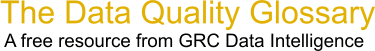Choose Index below for a list of all words and phrases defined in this glossary.

# Mathematical Expression

index | Index

## Mathematical Expression - definition(s)

mathematical expression - [mathematics] A kind of expression that evaluates to a number which is then typically stored in a variable, a field on a table row, or a cell in a raster dataset. Mathematical expressions are generally part of an algebraic equation:

Result = Expression

[PropertyTax] = ( [LandValue] * 0.75 ) + ( [StructureValue] * 0.50 )

[Category=Geospatial ]

Source: esri, 07 June 2012 12:47:14, http://support.esri.com/en/knowledgebase/GISDictionary/term/abbreviationData Quality Glossary.  A free resource from GRC Data Intelligence. For comments, questions or feedback: dqglossary@grcdi.nl